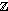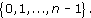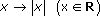Activity 7 Health care personnel in Thailand: calculating percentages

Would it be helpful, in considering possible changes in the way health care personnel are divided into the five categories listed, to recalculate th
Author(s): The Open University

There are practically no new theories or new principles in this section. We shall work through some examples, and you will see how basic techniques and approaches that you have already learned can be combined to allow you to use tabular data efficiently.

Example 3.1 Health personnel in Thailand

Author(s): The Open University

Activity 2 Boxplots of family sizes

The table below contains data on the sizes (numbers of children) of the completed families of two samples of mothers in Ontario. One sample of mothers had had fewer years of educatio
Author(s): The Open University

For the 23 infants who survived SIRDS, the ordered birth weights are given in Table 9. The first quartile is

qL = x (¼(23+1)) = x (6) = 1.720kg.

The third quartile is

qU = x
Author(s): The Open University

The first alternative measure of dispersion we shall discuss is the interquartile range: this is the difference between summary measures known as the lower and upper quartiles. The quartiles are simple in concept: if the median is regarded as the middle data point, so that it splits the data in half, the quartiles similarly split the data into quarters. This is, of course, an over-simplification. With an even number of data points, the median is defined to be the average of the middle two: de
Author(s): The Open University

The second measure of location defined in this course for a collection of data is the mean. Again, to be precise, we are discussing the sample mean, as opposed to the population mean. This is what most individuals would understand by the word ‘average’. All the items in the data set are added together, giving the sample total. This total is divided by the number of items (the sample size).

Author(s): The Open University

The data in Table 3 are the birth weights (in kg) of 50 infants suffering from severe idiopathic respiratory distress syndrome. There are two groups of infants: those who survived the condition (there were 23 of these) and those who, unfortunately, did not. The data have not been sor
Author(s): The Open University

Activity 21

Without using your calculator solve the following calculations.

• (a) 3 + 5 × 2 = ?

• (b) 12 − 6 + 6 = ?

• (c) 6 + (5 +
Author(s): The Open University

When calculating an answer it is important that you give careful consideration to the order of operations used in the calculation. If you are using a mixture of operations remember that certain operations take priority in a calculation. Consider the following, apparently, simple sum.

1 + 2 × 3 = ?

What answer would you give?

Did you give 7 as your response, or 9?

The correct answer is 7 but can you explain why?

If you have a calculator handy, check that it
Author(s): The Open University

After studying this course, you should be able to:

• round a given whole number to the nearest 10, 100, 1000 and so on

• round a decimal number to a given number of decimal places or significant figures

• use rounded numbers to find rough estimates for calculations

• use a calculator for decimal calculations involving +, −, × and ÷, giving the answer to a specified accuracy (e.g. decimal places or significant figures) and checking the ans
Author(s): The Open University

In the last subsection we stated that, for any integer n ≥ 2, the setn satisfies the same rules for addition modulo n as the real numbers satisfy for ordinary addition. When it comes to multiplicat
Author(s): The Open University

The Division Algorithm tells us that all the possible remainders on division by an integer n lie in the setWe denote this set by [Image_Link]https://www.open.edu/
Author(s):
The Open University

In OpenLearn course Diagrams, graphs and charts you saw how a scale is used on plans of houses and other structures. The scale makes it possible to take a length on the plan and calculate the correspond
Author(s): The Open University

Two straight lines that do not intersect, no matter how far they are extended, are said to be parallel. Arrows are used to indicate parallel lines.Author(s): The Open University

Question 1

Calculate all the angles at the centres of these objects.

Here is an improved solution which shows working.

Example 13

Suppose you plan to redecorate your bathroom. The end wall has the following shape, with dimensions as shown on the diagram. The quality of the plasterwork is not good and you are considering tiling the wall.

Author(s): The Open University

Example 14

Suppose you have decided to tile the wall using square tiles of side 10 cm. You are proposing to use the tiles across the full 5 metre width of the wall up to a height of 1.8 m.

Find the number of boxes of tiles that you will require to cover the wall if the tiles a
Author(s): The Open University

The modulus function provides us with a measure of distance that turns the set of complex numbers into a metric space in much the same way as does the modulus function defined on R. From the point of view of analysis the importance of this is that we can talk of the closeness of two complex numbers. We can then define the limit of a sequence of complex numbers in a way which is almost identical to the definition of the limit of a real sequence. Another analogue of real analysis arises
Author(s): The Open University

You will no doubt recall that in real analysis extensive use is made of the modulus function. It gives us a way of measuring the “closeness” of two numbers, which we exploit in writing expressi
Author(s): The Open University